Function Repository Resource:

# CompoundScope

Scope with compounding expressions

Contributed by: Nikolay Murzin
 ResourceFunction["CompoundScope"][{x=x0,y=y0,…},expr] specifies that all occurences of the symbols x,y,… in expr should be replaced by values x0,y0,… where each value can depend on all the previous values. ResourceFunction["CompoundScope"][{x=x0,code,y=y0,…},expr] also evaluates code that may depend on previously assigned values. ResourceFunction["CompoundScope"][x=x0;code;y=y0;…,expr] is the same as ResourceFunction["CompoundScope"][{x=x0,code,y=y0,…},expr]. ResourceFunction["CompoundScope"][scope,assignments,expr] applies a different scoping construct scope, which can be either With,Block or Module.

## Details

ResourceFunction["CompoundScope"][values,expr] is equivalent to ResourceFunction["CompoundScope"][With,values,expr].
ResourceFunction["CompoundScope"] recursively uses the specified scoping construct for each individual value assignment.

## Examples

### Basic Examples (2)

Evaluate an expression with x locally set to 7 and y to its square:

 In:=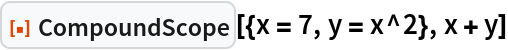Out=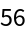Echo an intermediate assigned value:

 In:=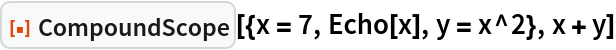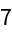Out=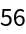### Scope (2)

Use CompoundExpression instead of a List to separate assignments:

 In:=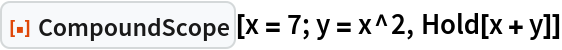Out=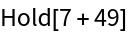Use different scoping functions:

 In:=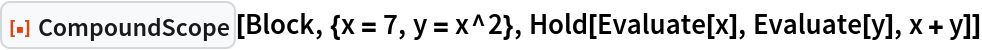Out=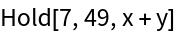Using Module introduces module variables:

 In:=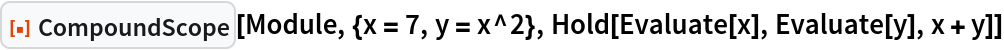Out=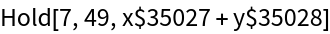### Properties and Relations (2)

Standard scoping constructs do not allow values to depend on previously assigned values:

 In:=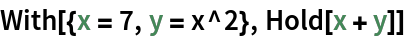Out=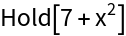CompoundScope does compound assignments:

 In:=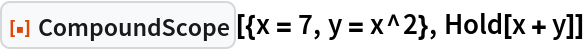Out=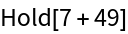## Version History

• 1.0.0 – 25 October 2022